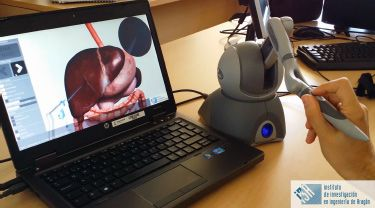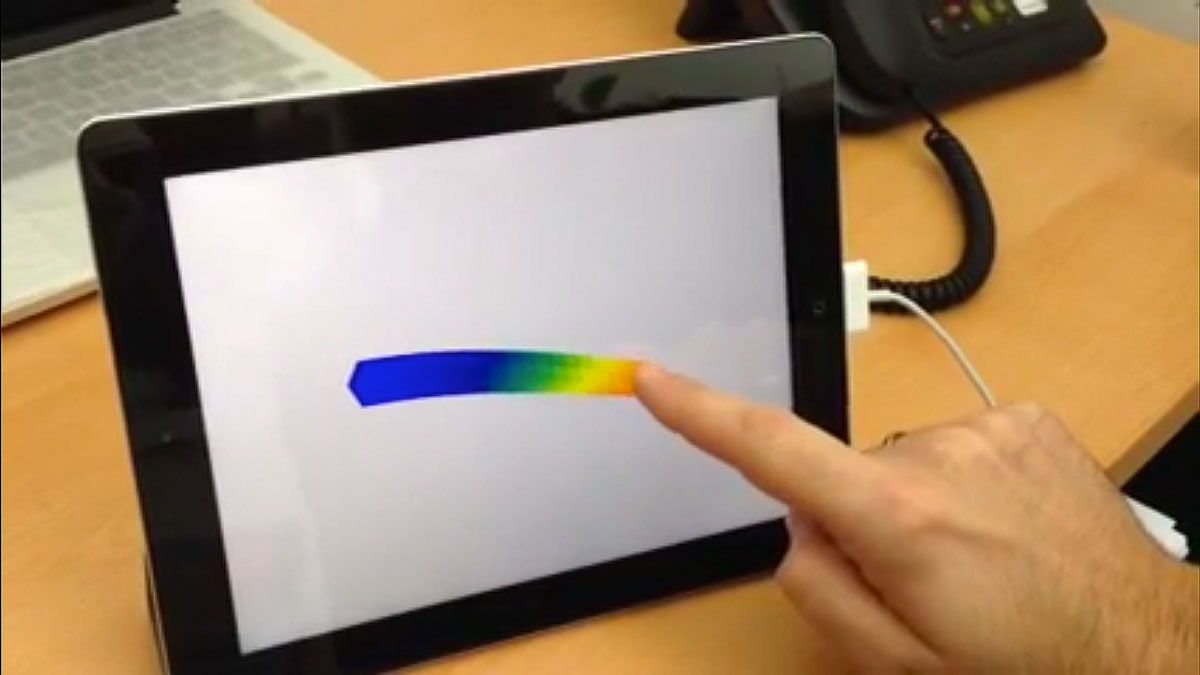# Proper Generalized Decomposition in MATLAB for Real-Time 3D Applications

By Dr. David González, University of Zaragoza (UniZar)

Most scientists, researchers, and engineers who perform structural analysis at some point encounter the curse of dimensionality: the inability to incorporate algorithms based on finite elements to real-time applications, given the dimensionality of the computation and performance requirements. Model order reduction (MOR) techniques such as proper orthogonal decomposition (POD) attempt to address the problem by reducing model complexity. These techniques are not suitable for real-time applications, however, because they are computationally costly.

I worked with two colleagues, Professors Elías Cueto and Icíar Alfaro, at the Aragon Institute of Engineering Research at the University of Zaragoza (UniZar) to develop a novel model order reduction approach based on proper generalized decomposition (PGD)1. Implemented in MATLAB®, this approach incorporates two phases: an offline phase in which a multiparametric solution to the problem is computed, and an online phase in which the solution computed in the first phase is used to obtain results in real time.

PGD has a number of applications across a variety of domains. For example, it can be used to calculate surface deformation in response to a moving applied load—a problem long considered nonreducible for real-time applications. To demonstrate our implementation of PGD, we’ve developed several sample applications, including an iPad app that simulates the deformation of a cantilever beam in real time when a load is applied, and a real-time surgical simulator with haptic feedback that enables the operator to see and feel the response of a virtual liver to various simulated loads directed via a stylus (Figure 1).Figure 1. Real-time liver palpation simulation powered by a MATLAB based PGD implementation.

## Implementing a PGD Framework in MATLAB

The computer-aided engineering (CAE) community has been working on model order reduction for decades. The history of PGD began with the LATIN multiscale computational method. Over the past 10 years, Professor Francisco Chinesta and his team have used PGD and MATLAB to develop models of non-Newtonian fluids. When my colleagues and I began working with PGD, we built upon many of the concepts and techniques that he developed, and we, too, selected MATLAB for the implementation of our PGD framework for dynamic problems.

I had programmed extensively in C++ for my Ph.D., but switched to MATLAB for this project because PGD requires numerous vector and matrix manipulations, and MATLAB enabled me to write efficient, vectorized code for these operations. Minimizing the use of `for` loops made the code execute faster and made it easier to write and maintain. Code performance was a priority because the preprocessing required for the initial multiparametric solution is computationally intensive and can take several hours to complete.

The results of the first phase of PGD calculations are stored as a large, multidimensional array in the MATLAB workspace (Figure 2). We then either postprocess and visualize the data directly in MATLAB or export it in one of a variety of formats for use in 3D visualization software and other custom applications.

One of the custom apps that we developed was an iPad app that simulates the deformation of a cantilever beam in real time when a load is applied (Figure 3). This app complements mechanical engineering theory by helping students understand the basics through experimentation.Figure 3. Interactive iPad app simulating a cantilever beam bending in response to a load applied at the point where the user’s finger contacts the screen.

## Packaging PGD as a MATLAB App

To make the PGD framework easier for students and researchers to use and experiment with, we created a shareable MATLAB app (Figure 4). The student or researcher begins by selecting a preset use case, such as the cantilever beam, the liver, or similar examples for the pancreas and gall bladder. The user can specify whether the underlying material behavior should be treated as elastic or hyperelastic and whether the problem should be solved using a static approach or a dynamic approach in which the material's response at any timestep is partially determined by its response in the previous timestep.

The app uses PGD to compute the solution, which is then available in the MATLAB workspace.  The solution for any problem needs to be computed only once: The results of the initial phase can be saved as a MAT-file and recalled for postprocessing or visualization (Figure 5).

I use the MATLAB app to introduce PGD concepts to students in two master's-level classes: Numerical Methods in Bioengineering and Computational Methods in Engineering. Because the coursework in both classes requires the students to use MATLAB, I begin each course with a short review of MATLAB basics. Students in these classes not only learn to develop and share numerical and computational methods, they also develop an understanding of PGD and how to apply it using MATLAB.

## Case Study: Creating a Real-Time Simulation with Haptic Feedback for Liver Palpation

One of my principal research areas is the application of computing, simulation, and imaging to surgical procedures. The surgical simulator shown in Figure 1 is one example of the value of PGD in this area. To create it, we used our MATLAB PGD framework to compute a multiparametric solution using three input parameters: a 3D mesh of the liver, a set of all possible positions for the loads (points where the surgeon could apply force to the liver), and the activation of the load from 0% (no load) to 100% (full load). We then worked with a team of programmers to incorporate the computed result matrix into a simulator with real-time visualization and haptic feedback.

During a simulation, any movement of the manual stylus causes a corresponding movement of the virtual stylus on screen. When the operator presses a button on the stylus, the virtual stylus is pressed against the simulated liver. The liver surface's reaction to this pressure is calculated using the computed PGD results. Because this calculation only requires straightforward vector multiplication, it can be completed hundreds of times per second to support real-time performance. The resulting deformation of the liver is shown on screen, and an appropriate signal is sent to the haptic device, enabling the operator to feel resistance on the stylus in real time.

After calibrating and tuning the simulator, we had it tested by experienced surgeons. They reported that the sensation they felt through the haptic device authentically reproduced the sensation of working on an actual patient.

We recently updated the simulator to support patient-specific liver geometries. In this version of the simulator, the patient’s liver is first imaged to create a 3D model. The model is then compared against a library of liver geometries with PGD solutions that have already been computed. The computed solution for the library model most similar to that of the patient is then used in the simulation.

With this capability, together with a planned enhancement that will support tearing and cutting operations, we will be able to create a realistic simulator for surgical training. This simulator will make it possible for medical students to practice safely and for experienced surgeons to rehearse surgical procedures on lifelike virtual organs before performing the actual procedures in the operating room.

1 Compared with dynamics simulation using finite element analysis (FEA), PGD reduces the degree of freedom (DOF) by projecting the nonlinear element basis equations onto a set of parametrically reduced basis equations in the initial displacement field and again at each time step.